# Refraction Through A Prism Made Easy To Ace NEET

###### BySafeer PP###### Synopsis

Optics is an important part of the question paper for the National Eligibility cum Entrance Test (NEET), the gateway to medical admissions in the country. In the last five years' papers, there were 22 questions from the Optics Unit. Out of these 11 questions were from Ray Optics. Out of this four questions were from the topic Refraction Through A Prism, an important topic for JEE Main too. Read on to know the concept of Refraction Through A Prism And The NEET questions based on this topic.###### Synopsis

Optics is an important part of the question paper for the National Eligibility cum Entrance Test (NEET), the gateway to medical admissions in the country. In the last five years' papers, there were 22 questions from the Optics Unit. Out of these 11 questions were from Ray Optics. Out of this four questions were from the topic Refraction Through A Prism, an important topic for JEE Main too. Read on to know the concept of Refraction Through A Prism And The NEET questions based on this topic.

The unit Optics has two chapters namely. Ray Optics And Optical Instruments; and Wave Optics. The unit Optics is important for the NEET UG exam as 22 questions were asked from the unit in the last five years’ papers (2017 to 2021). Out of these 22 questions, 11 were from the chapter Ray Optics And Optical Instruments and 11 from Wave Optics. Four questions out of 11 from Ray Optics were from the concept of Refraction Through A Prism. So, Refraction Through A Prism is an important topic for NEET. At the same time, JEE Main Papers also has a number of questions from the concept of Refraction Through A Prism. So, preparing this topic, that is, Refraction Through A Prism, is important for you to score well in both these entrance exams, NEET and JEE Main.

## Refraction Through A Prism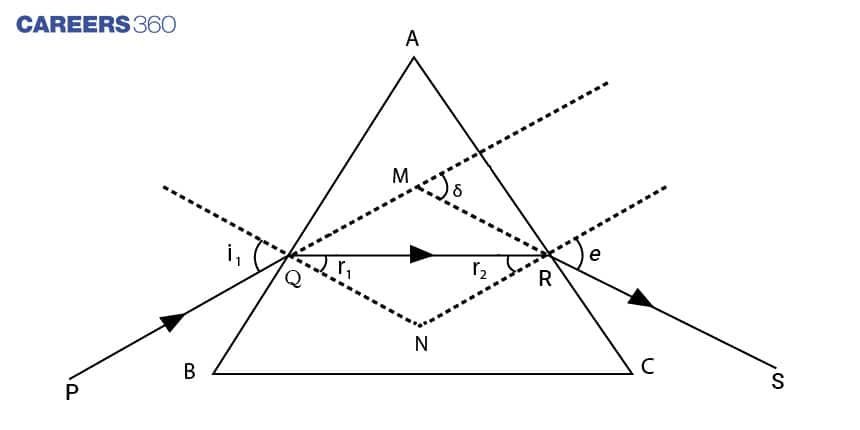Consider a triangular prism of refractive index n. It has two triangular bases and three rectangular lateral surfaces. These surfaces are inclined to each other. The angle between its two lateral faces is called the angle of the prism A .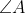is the angle included between the two refracting faces of the prism.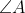is also known as refracting angle. i and r1 are angles of incidence and refraction respectively at face AB. r2 and e are angles of incidence and refraction respectively at face AC. e is also called the angle of emergence.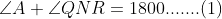From triangle QNR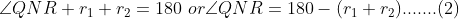From (1) and (2)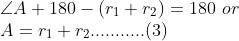### Angle Of Deviation (δ)

The angle between the ray of incidence (PQ) and emergence (RS) is known as the angle of deviation.

The angle of deviation δ=sum of deviations at two refracting faces. That is

δ=(i-r1)+(e-r2)=i+e-A……(4)

Question JEE Main 2017

A ray of light is incident at an angle of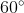on one face of a prism of angle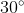. The emergent ray of light makes an angle ofwith incident ray. The angle made by the emergent ray with the second face of the prism will be:

Solution:

Given δ=300, i=600 and A=300

δ=i+e-A

e=δ+A-i=30+30-60=00. The angle made with the second face is=900. So the answer is 90

Note-The angle e is with respect to the normal. There is a greater chance to mark the answer as zero which is wrong. Properly read the question to avoid this type of error.

### Angle Of Minimum Deviation (δmin)

The deviation angle is minimum when i=e. When i=e, r1=r2=r. That refracted ray inside the prism is parallel to the base.

From equation (3)

A=2r or r=A/2

And from equation (4)

δmin=2i-A

i=(A+δmin)/2

Now apply the snell's law at face AB. If n is the refractive index of the prism. Let the prism be placed in the air(refractive index of the air taken as 1), then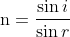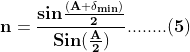Equation (5) is the relation between the refractive index and the minimum angle of deviation of the prism.

For a thin prism, δmin and angle A is very small. Therefore

δmin=(n-1)A………(6)

Question: JEE Main 2019

Monochromatic light is incident at a certain angle on an equilateral triangular prism and suffers minimum deviation. If the refractive index of the material of the prism is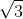, then the angle of incidence is:

Solution:

Since the given prism is an equilateral prism, A=600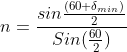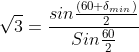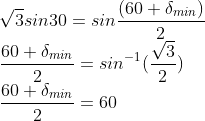δmin=600

i=(A+δmin)/2=(60+60)/2=600. The angle of incidence is 600

### The Plot Of Angle Of Deviation ( δ) Versus Angle Of Incidence (i)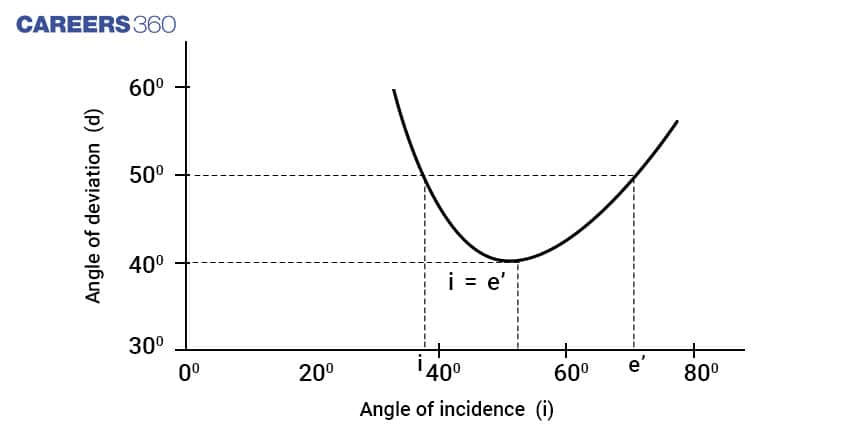From the graph, it is clear that for deviation angles other than the minimum deviation, there are two values of i and hence for e. This implies that δ remains the same if i and e are interchanged. Let us see previous year's NEET questions based on the topic Refraction Through A Prism.

## Questions From Previous 5 Year NEET Paper

Question: NEET 2017

A thin prism having refracting angle 10° is made of glass of refractive index 1.42. This prism is combined with another thin prism of glass of refractive index 1.7. This combination produces dispersion without deviation. The refracting angle of the second prism should be:

Solution:

Note: For Dispersion without deviation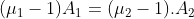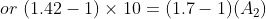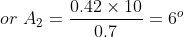Question: NEET 2018

The refractive index of the material of a prism is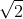and the angle of the prism is 30o. One of the two refracting surfaces of the prism is made a mirror inwards, with a silver coating. A beam of monochromatic light entering the prism from the other face will retrace its path (after reflection from the silvered surface) if its angle of incidence on the prism is

Solution:

Here r2=0

Therefore from the relation A=r1+r2, r1=A=300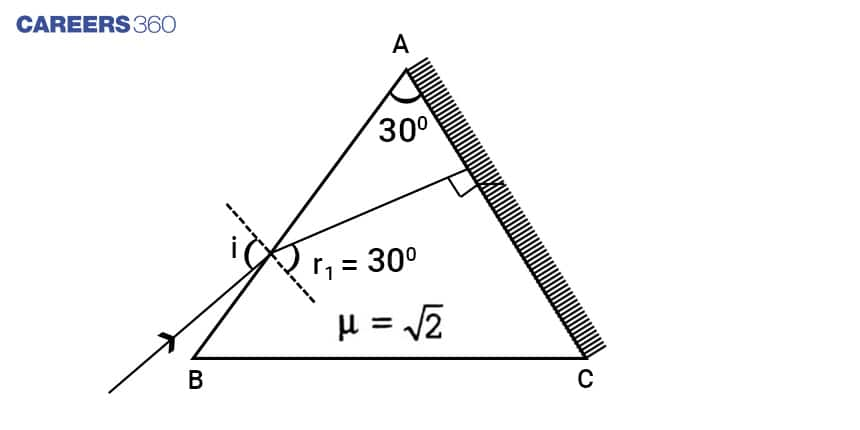Applying snell's law at face AB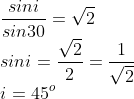450 is the angle for which the beam retraces its path.

Question: NEET 2020

A ray is incident at an angle of incidence i on one surface of a small angle prism (with the angle of prism A) and emerges normally from the opposite surface. If the refractive index of the material of the prism is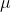then the angle of incidence is nearly equal to:

Solution: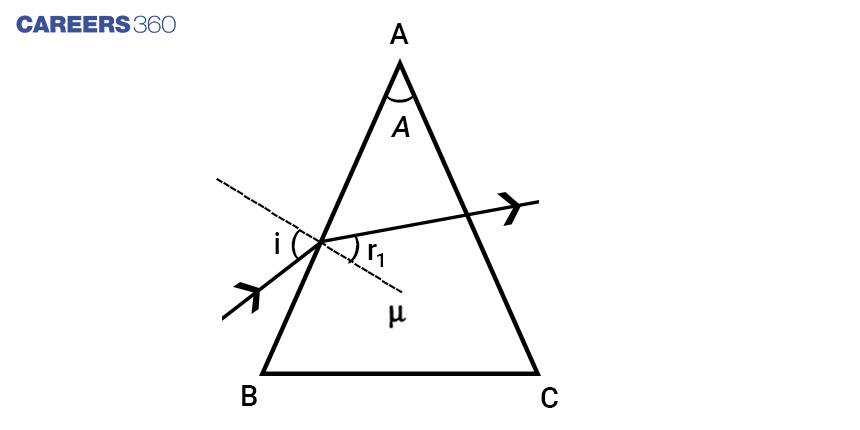Given that ray emerges normally from the opposite surface. This implies that e=0 . Also r2=0(angle of incidence at AC is zero, so angle of refraction at face AC is zero(e=0)). Therefore from A=r1+r2

A=r1

Therefore applying snell's law for face AB

sini/sinA=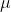Since it is thin prism i=AQuestion: NEET 2021

Find the value of the angle of emergence from the prism. The Refractive index of the glass is.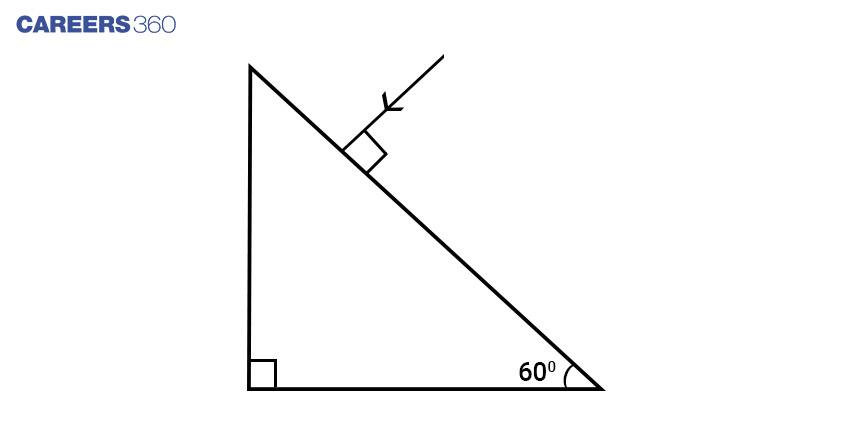Solution: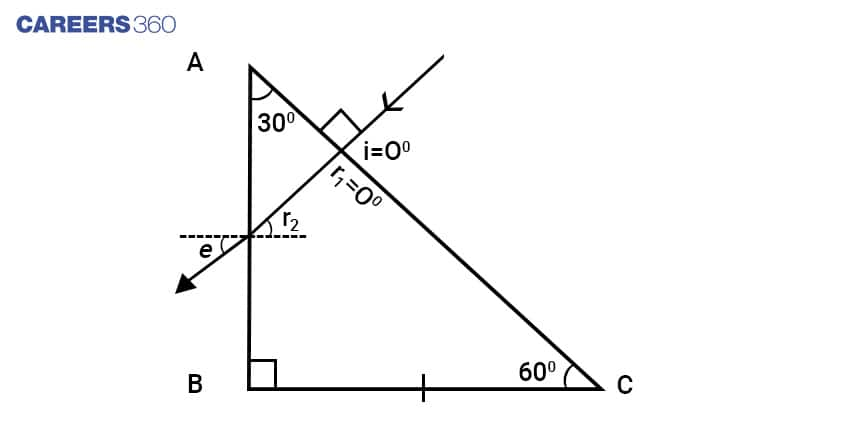r1=0 as i=0

Therefore A=r2 (since A=r1+r2)

Applying snell's law at AB

sine/sinA=sine=/2

e=60o

If we look at the past five years’ papers, except 2019, all other papers have one question from the topic – Refraction Through A Prism. Eleven questions were asked from Ray Optics and out of these 4 questions were from the topic Refraction Through A Prism. That means, 36.36% of questions from Ray Optics are related to Refraction Through A Prism, which makes it an important topic. Refraction Through A Prism is a small topic and can be understood easily. To solve problems, students need to understand the Snell's Law properly and how to apply it in solving problems.

• Physics
• Electromagnetism
• Optics
• Physical chemistry
• Applied and interdisciplinary physics
• Force
• Atomic, molecular, and optical physics
• Natural philosophy
• Physical phenomena

## Careers360 helping shape your Career for a better tomorrow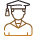#### 250M+

Students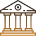#### 30,000+

Colleges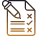#### 500+

Exams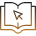E-Books